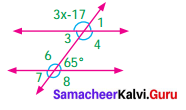Students can Download Maths Chapter 5 Geometry Ex 5.2 Questions and Answers, Notes Pdf, Samacheer Kalvi 7th Maths Book Solutions Guide Pdf helps you to revise the complete Tamilnadu State Board New Syllabus and score more marks in your examinations.

## Tamilnadu Samacheer Kalvi 7th Maths Solutions Term 1 Chapter 5 Geometry Ex 5.2

Question 1.
From the figures name pair of angles.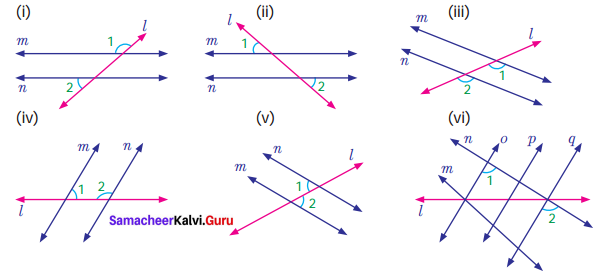Solution:
(i) m and n are parallel lines and l is the transversal.
∴ ∠1 and ∠2 are exterior angles on the same side of the transversal.

(ii) m and n are parallel lines and l is the transversal
∠1 and ∠2 are alternate exterior angles.

(iii) m and n are parallel lines l is the transversal
∠1 and ∠2 are corresponding angles.

(iv) m and n are parallel lines l is the transversal.
∠1 and ∠2 are interior angles on the same side of the transversal.

(v) m and n are parallel lines and l is the transversal.
∠1 and ∠2 are alternate interior angles.

(vi) o and q are parallel lines and n is the transversal.
∠1 and ∠2 are corresponding angles.Question 2.
Find the measure of angle x in each of the following figures.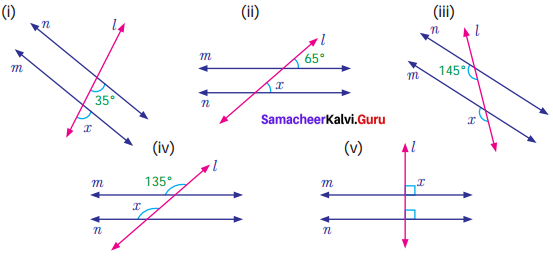Solution:
(i) m and n are parallel lines and l is the transversal.
x° and 35° are corresponding angles and so they are equal.
∴ x = 35°

(ii) m and n are parallel lines and l is the transversal.
∴ x = 65°
[∴ corresponding angles are equal].

(iii) n and m are parallel lines and l is the transversal.
Corresponding angles are equal
∴ x = 145°

(iv) m and n are parallel lines and l is the transversal.
Corresponding angles are equal
∴ x = 135°

(v) m and n are parallel lines, l is the transversal perpendicular to both the lines
∴ x = 90°Question 3.
Find the measure of angles in each of the following figures.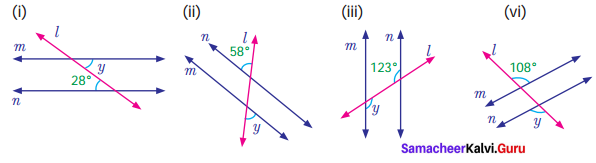Solution:
(i) m and n are parallel lines. l is the transversal. Then alternate interior angles are equal
∴ y = 28°

(ii) m and n are parallel lines. l is the transversal. Alternate exterior angles are equal
∴ y = 58°

(iii) m and n are parallel lines. l is the transversal.
Alternate interior angles are equal
∴ y = 123°

(iv) m and n are parallel lines . l is the transversal
alternate exterior angles are equal.
∴ y = 108°Question 4.
Find the measure of angle z in each of the following figures.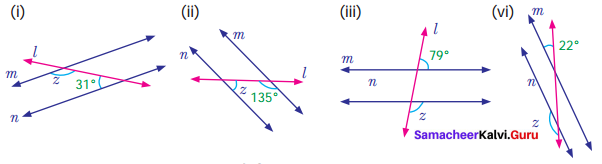Solution:
(i) m and n are parallel lines l is the transversal
Then interior angles that lie on the same side of the transversal are supplementary
∴ z + 31° = 180°
z + 31° – 31° = 180° – 31°
z = 149°

(ii) m and n are parallel lines, l is the transversal
Interior angles that lie on the same side of the transversal are supplementary
∴ z + 135° = 180°
z + 135° – 135° = 180° – 135°
z = 45°

(iii) m and n are parallel lines l is the transversal exterior angles that lie on the same side of the transversal are supplementary.
∴ z + 79° = 80°
z + 79° – 79° = 180° – 79°
z = 101°

(iv) m and n are parallel lines and l is the transversal. Corresponding angles are equal
z + 22° = 180°
z + 22° – 22° = 180° – 22°
z = 158°Question 5.
Find the value of angle ‘a’ in each of the following figures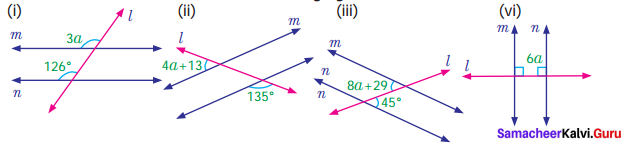Solution:
(i) m and n are parallel lines. l is the transversal
∴ Corresponding angles are equal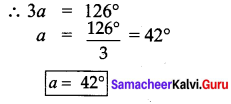(ii) m and n are parallel lines l is the transversal
Exterior angles that lie on the same side of the transversal are supplementary
∴ (4a + 13) + 135° = 180°
4a + 13 + 135° = 180°
4a +148° = 180°
4a + 148° – 148° = 180° – 148°
4a = 32°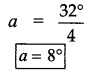(iii) m and n are parallel lines l is the transversal
∴ Alternate interior angles are equal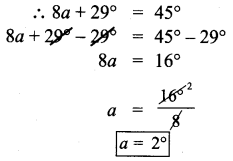(iv) m and n are parallel lines l is the transversal which is perpendicular to m and n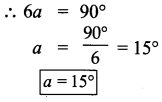Question 6.
Find the value of the angle x in both the figures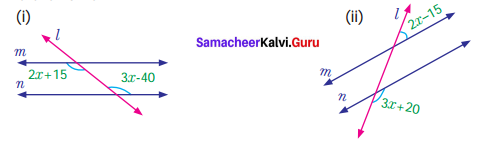Solution:
(i) m and n are parallel lines. l is the transversal
∴ Alternate interior angles are equal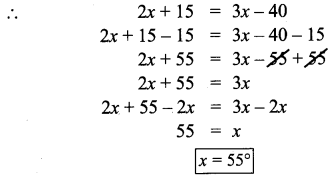(ii) m and n are parallel lines. l is the transversal
Exterior angles on the same side of the transversal are supplementary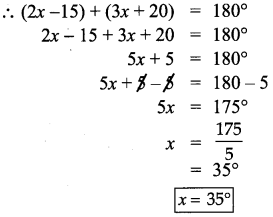Question 7.
Anbu has marked the angles as shown below in (i) and (ii). Check whether both of them are correct. Give reasons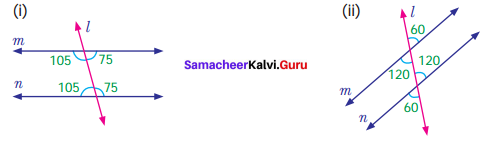Solution:
(i) m and n are parallel lines. l is the transversal.
Interior angles on the same side of the transversal are supplementary. But here it is 75 + 75 ≠ 180°
105 + 105 ≠ 180°
∴ Angles marked are not correct

(ii) m and n are parallel lines. l is the transversal.
Corresponding angles must be equal. So here the marking is wrong.

Question 8.
Mention two real life situations where we use parallel lines.
Solution:
Two angles of a wall in a building Cross rods in a window.

Question 9.
Two parallel lines are intersected by a transversal. What is the minimum number of angles you need to know to find the remaining angles. Give reasons.
Solution: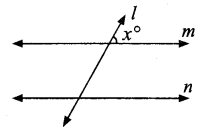When two parallel lines are intersected by a transversal, we need a minimum of a single angle to find the remaining angle.
Using the concept of linear pair of angles, we can final one more angle.
By the concepts of corresponding angles, alternate interior angles and alternate , exterior angles we could find all other angles.

Objective Type Questions

Question 10.
A line which intersects two or more lines in different points is known as
(i) parallel lines
(ii) transversal
(iii) non-parallel lines
(iv) Intersecting lines
Solution:
(ii) Transversal

Question 11.
In the given figure angles a and b are
(i) alternate exterior angles
(ii) corresponding angles
(iii) Alternate interior angles
(iv) Vertically opposite angles
Solution:
(i) alternate exterior angles

Question 12.
Which of the following statement is ALWAYS TRUE when parallel lines are cut by a transversal
(i) corresponding angles supplementary
(ii) alternate interior angles supplementary
(iii) alternate exterior angles supplementary
(iv) interior angles on the same side of the transversal are supplementary
Solution:
(iv) Interior angles on the same side of the transversal are
supplementary.

Question 13.
In the diagram what is the value of angle x?
(i) 43°
(ii) 44°
(iii) 132°
(iv) 134°
Hint: